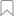## FaceAnalysisDa PromityAI | Aggiornamento 2 mesi fa | Visual Recognition
Popolarità

Latenza

## 1,638ms

Livello di servizio

## 95%

Torna a tutti i tutorial (4)

# Prerequists

For this tutorial you would need to install these python packages:

• numpy
• opencv
• requests

You can install it by pip:
`pip install numpy opencv-python requests`
or
`pip3 install numpy opencv-python requests`

# Send image by URL

First of all we have to import required packages:

``````import cv2
import json
import requests
import numpy as np
``````

Next let’s define some constants:

``````img_address = "https://promity.com/wp-content/uploads/2021/05/image-0111a.jpg"
api_key = "XXX"
``````

Where:

• img_address is URL address of image that you want to process. In this case we provided here some random image from Internet,
• api_key is your key from RapidAPI,

Now we can send request to API:

``````headers = {
"X-Rapidapi-Key": api_key,
"Content-Type": "application/json",
}
``````

and convert response from json format to ‘easier to use’ dictionary:

``````response_dict = json.loads(response.text)
``````

Now, let’s make some visualization. At first we have to download our image from Internet:

``````resp_img = requests.get(img_address, stream=True)
arr = np.asarray(bytearray(resp_img.content), dtype=np.uint8)
img = cv2.imdecode(arr, -1)
``````

Get height and width of image, which will be needed later:

``````img_height, img_width, _ = img.shape
``````

Now iterate through all detections and put bounding boxes around detected faces:

``````for det in response_dict['detections']:
crop = det['crop']
if crop['score'] < 0.8:
continue
x1 = int(crop['x1'] * img_width)
x2 = int(crop['x2'] * img_width)
y1 = int(crop['y1'] * img_height)
y2 = int(crop['y2'] * img_height)
img = cv2.rectangle(img, (x1, y1), (x2, y2), (0, 255, 0), 3)
``````

Value of crop[‘score’] tells us, how face detection algorithm was sure, that there is face in given region. Maximum of this value is 1.0, which means that our algorithm was 100% sure. In other words, it’s probability. In this example, if it’s less than 0.8, we ommit this detection.
OK, let’s look on our image:

``````cv2.imshow('Promity Rapidapi example', img)
cv2.waitKey(0)
cv2.destroyAllWindows()
``````

Or save it to disk:

``````cv2.imwrite('promity_face_detection.png', img)
``````

## Full code

``````import cv2
import json
import requests
import numpy as np

api_key = "XXX"

"X-Rapidapi-Key": api_key,
"Content-Type": "application/json",
}

print(response.text)

arr = np.asarray(bytearray(resp_img.content), dtype=np.uint8)
img = cv2.imdecode(arr, -1)

img_height, img_width, _ = img.shape

for det in response_dict['detections']:
crop = det['crop']
if crop['score'] < 0.8:
continue
x1 = int(crop['x1'] * img_width)
x2 = int(crop['x2'] * img_width)
y1 = int(crop['y1'] * img_height)
y2 = int(crop['y2'] * img_height)
img = cv2.rectangle(img, (x1, y1), (x2, y2), (0, 255, 0), 3)
cv2.imshow('Promity Rapidapi example', img)
cv2.waitKey(0)
cv2.destroyAllWindows()
cv2.imwrite('promity_face_detection.png', img)
``````

# Send image from your local system

First of all we have to import required packages:

``````import cv2
import json
import requests
``````

Next let’s define some contants:

``````img_path = 'example_image.jpg'
api_key = "XXX"
``````

Where:

• img_path is a path to image in your local system. We will send this image to face detection API.
• api_key is your key from RapidAPI
Now we can send request to API:
``````files = {'image_file': open(img_path, 'rb')}
"x-rapidapi-key": api_key
}
``````

and convert response from json response to dictionary:

``````json_dict = json.loads(response.text)
``````

Now read image and get it’s height and width, which will be used during visualization process:

``````img = cv2.imread(img_path)
img_height, img_width, _ = img.shape
``````

Let’s iterate through all detections and put bounding boxes around detected faces:

``````for det in json_dict['detections']:
crop = det['crop']
if crop['score'] < 0.8:
continue
x1 = int(crop['x1'] * img_width)
x2 = int(crop['x2'] * img_width)
y1 = int(crop['y1'] * img_height)
y2 = int(crop['y2'] * img_height)
img = cv2.rectangle(img, (x1, y1), (x2, y2), (0,250,0), 3)
``````

Value of crop[‘score’] tells us, how face detection algorithm was sure, that there is face in given region. Maximum of this value is 1.0, which means that our algorithm was 100% sure. In other words, it’s probability. In this example, if it’s less than 0.8, we ommit this detection.
OK, let’s look on our image:

``````cv2.imshow('Promity Rapidapi example', img)
cv2.waitKey(0)
cv2.destroyAllWindows()
``````

Or save it to disk:

``````cv2.imwrite('promity_face_detection.png', img)
``````

## Full code

``````import cv2
import json
import requests

img_path = 'example_image.jpg'
api_key = "XXX"

files = {'image_file': open(img_path, 'rb')}
"x-rapidapi-key": api_key
}

img_height, img_width, _ = img.shape

for det in json_dict['detections']:
crop = det['crop']
if crop['score'] < 0.8:
continue
x1 = int(crop['x1'] * img_width)
x2 = int(crop['x2'] * img_width)
y1 = int(crop['y1'] * img_height)
y2 = int(crop['y2'] * img_height)
img = cv2.rectangle(img, (x1, y1), (x2, y2), (0,250,0), 3)

cv2.imshow('Promity Rapidapi example', img)
cv2.waitKey(0)
cv2.destroyAllWindows()

cv2.imwrite('promity_face_detection.png', img)
``````
Valutazione: 5 - Voti: 1